# Geomtery (Test 1)

## Cat Entrance Exams : Mathematics Or Quantitative Aptitude

| Home | | Cat Entrance Exams | | Mathematics Or Quantitative Aptitude | | Geomtery |Geomtery
| Geomtery |
Q.1
A triangle with one angle greater than 90 degrees.
A. equilateral triangle
B. obtuse triangle
C. acute triangle
D. isosceles triangle
Answer : Option A
Explaination / Solution:
No Explaination.

Workspace
Report
Q.2
What is the volume of this rectangular prism?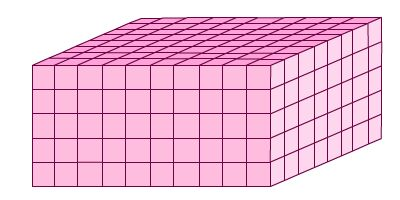A. 800 cubic units
B. 400 cubic units
C. 100 cubic units
D. 80 cubic units
Answer : Option B
Explaination / Solution:
No Explaination.

Workspace
Report
Q.3
What is the missing measurement for the line segment?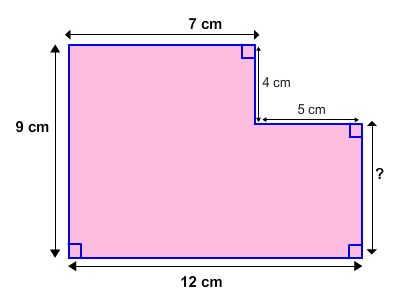A. 4 cm
B. 9 cm
C. 5 cm
D. 8 cm
Answer : Option C
Explaination / Solution:
No Explaination.

Workspace
Report
Q.4
What part of the area is shaded?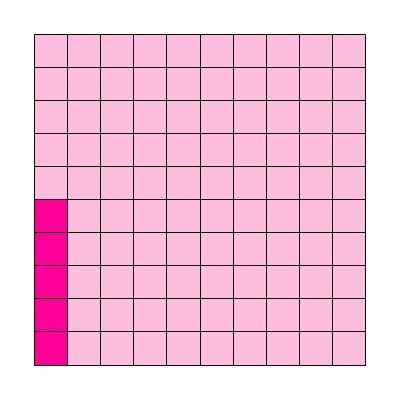A. 0.05
B. 0.5
C. 5.0
D. 0.005
Answer : Option A
Explaination / Solution:
No Explaination.

Workspace
Report
Q.5
Which ordered pair represents point R?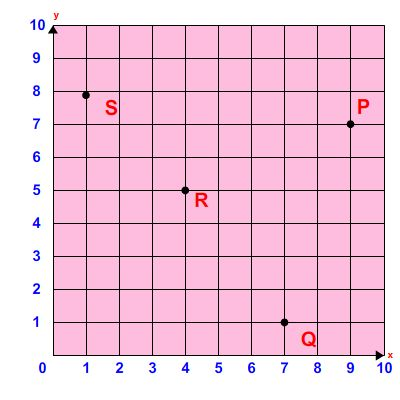A. (5,4)
B. (0,9)
C. (4,5)
D. (7,8)
Answer : Option C
Explaination / Solution:
No Explaination.

Workspace
Report
Q.6
What is the sum of the interior angles of rectangle below?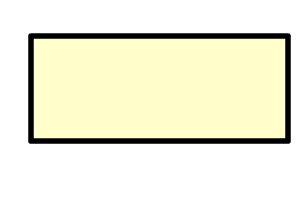A. 720 degrees
B. 360 degrees
C. 180 degrees
D. 520 degrees
Answer : Option B
Explaination / Solution:
No Explaination.

Workspace
Report
Q.7
which expresson shows the area of the rectangle?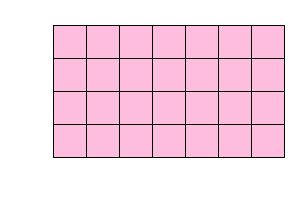A. 4 + 7
B. 4+7+4+7
C. 4 X 7
D. 4X7X4X7
Answer : Option C
Explaination / Solution:
No Explaination.

Workspace
Report
Q.8
Which ordered pair represents point P?A. (5,4)
B. (4,5)
C. (7,9)
D. (9,7)
Answer : Option D
Explaination / Solution:
No Explaination.

Workspace
Report
Q.9
Which ordered pair represents point R?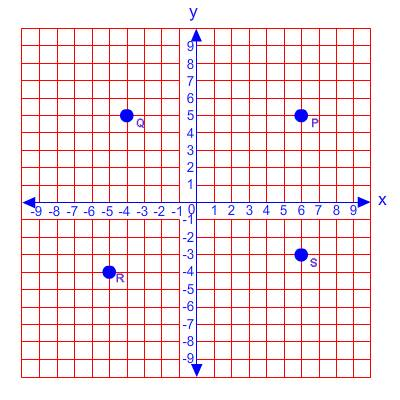A. (5,4)
B. (-5,-4)
C. (-4,-5)
D. (6,-3)
Answer : Option B
Explaination / Solution:
No Explaination.

Workspace
Report
Q.10
What is the best description of a point?
A. straight path that has no thickness and extends forever
B. names a location, has no size
C. flat surface that has no thickness and extends forever
Answer : Option B
Explaination / Solution:
No Explaination.

Workspace
Report